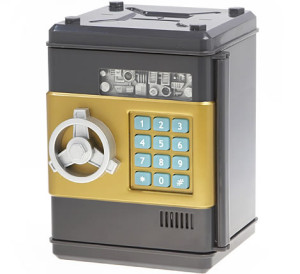# Safe key decrypt puzzle

1,076.8K Views

There is a safe with a 5 digit number as the key. The 4th digit is 4 greater than the second digit, while the 3rd digit is 3 less than the 2nd digit. The 1st digit is thrice the last digit. There are 3 pairs whose sum is 11.

Find the key to open the safe.let 5 digits be

a b c d e

now,

eq1=>    d= 4+b,
eq2=>    c = b- 3
eq3=>     a =3e,

As a,b,c,d,e are single digits can varies from 0-9

so
bmax <= 5 , from eq1
bmin >= 3, from eq

similarly
dmax = 9
dmin = 7

cmax = 2
cmin= 0

e can be     0, 1,2,3
a will be     0,3,6,9 respectively

So ranges are
0<=a<=9
3<=b<=5
0<=c<=2
7<=d<=9
0<=e<=3

Possible pairs are
ab, ac, ad, ae,     bc, bd, be,     cd, ce,      de

Also there are only three pairs whose sum should be 11
there are 9 cases lets find one by one
a.) if b= 3 => then  c = 0, d= 7
(i)  a = 9,  e= 3,
or  (ii) a = 6,  e = 2,
or (iii) a = 3, e =1

b.) if b = 4 => then c=1, d=8
(i)  a = 9,  e= 3,
or  (ii) a = 6,  e = 2,
or (iii) a = 3, e =1

c.) if b = 5 => then c=2, d= 9
(i)  a = 9,  e= 3,
or  (ii) a = 6,  e = 2,
or (iii) a = 3, e =1

So from this only c(ii) shows the desired result
a+b= 11, c+d =11, d+e = 11

a=6,b=5,c=2,d=9,e=2

ie. 65292